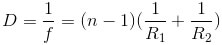﻿ Geometric Optics
﻿

## Laws Of Reflection

SI: incident ray, IR: reflected ray, i: angle of incidence, i’: angle of reflection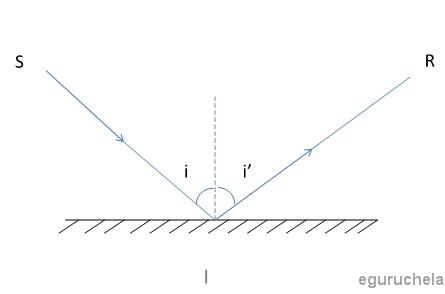## Refractive Index

n: refractive index of the medium, v: speed of light in a medium, c: speed of light in vacuum (m/s)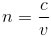## Law Of Refraction

n1: refractive index of the medium 1, n2: refractive index of medium 2, i: angle of incidence, r: angle of refraction, n21: absolute index of refraction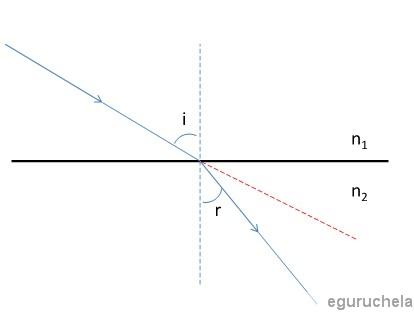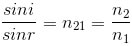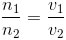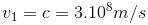## Total Reflection Phenomenon

There is no refracted ray if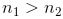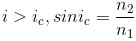## Prism

A: apex angle, D: deviation angle, i1: angle of incidence, i2: angle of emergent ray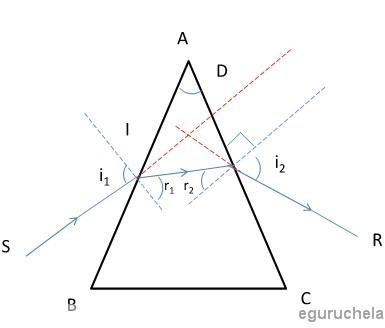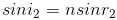Condition to have refractive rayWhen angle of incidence is minimum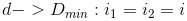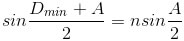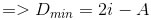## Thin Lens

f: focal length (converging len f>0; diverging len f<0), d: object distance from lens center, d’: image distance from lens center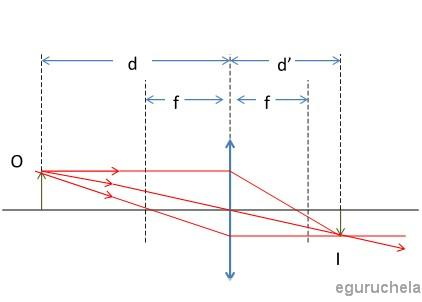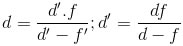## Magnification Factor

hI ; image height, ho: object height## Power Of The Lens

n: refractive index of the lens material, R1: radius of curvature of the lens surface closest to the light source, R2: radius of curvature of the lens surface farthest from the light source